# APL – solving Euler problem 1

Note: see the rest of the series so far.

Surprisingly, my last post on APL did well on HackerNews, turns out there is genuine interest in APL out there, which is great to seeI have been busy with a number of conferences and talks since then so I haven’t had time to revisit APL. Over the next couple of posts in this series (or rather, what I hope would amount to a series) let’s try to solve some simple problems with APL. Mind you, my knowledge of APL is pretty shallow so what I end up with is likely far from idiomatic APL code, so feel free to offer pointers/feedbacks in the comments section below!

To start us off nice and easy, let’s look at something really simple, like Euler Problem 1.

If we list all the natural numbers below 10 that are multiples of 3 or 5, we get 3, 5, 6 and 9. The sum of these multiples is 23.

Find the sum of all the multiples of 3 or 5 below 1000.

Super easy, right? I knew how to solve this with a one-liner even when I was a total beginner in F#.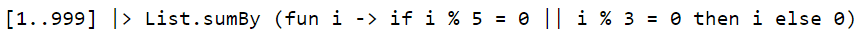which roughly translates to the following in APL: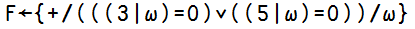$latex F \ \iota 9$ => 23

Looks great, and running $latex F \ \iota 999$ gives the right answer (I won’t spoil the answer for you, but feel free to run this in TryAPL at your own discretion!) So here’s what we did (p.s. | is the remainder operator):

1. find elements from the input $latex \omega$ that are divisible by 3 $latex (3|\omega)=0$ or 5 $latex (5|\omega)=0$
2. which gives us an array of booleans, which we then OR $latex \lor$
3. then we use compression to filter the input array $latex / \omega$ to return only the elements that are divisible by 3 or 5
4. and finally reduce over these elements with addition

p.s. OK, it’s not exactly a faithful translation of the F# code above, the equivalent F# code should probably use reduce as well: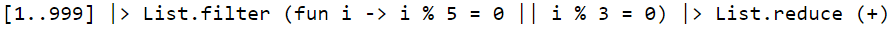UPDATE 24/07/2015 : much to my pleasant surprise this piqued Tomas’s interest and he even did a quick AplMode to show how you can mimic the APL code above in F# and solve the problem in 19 characters.

To be fair, I’m writing APL whilst thinking like a F#er so I’m not doing it justice, but Stefano shared an even shorter APL solution (17 characters):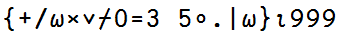This took me a little while to understand, but fortunately Dyalog APL‘s IDE’s really quite easy to use and so after some experimentation here’s how Stefano’s solution works:

(first you need to understand that in APL, you always read from RIGHT-TO-LEFT unless you can ( ))

1) generate an outer product ($latex \circ .$) of reminders (using the residue | operator):

• the array of 3 and 5; and
• input array $latex \omega$

this generates (for 1 to 9) the matrix:

1  2  0  1  2  0  1  2  0

1  2  3  4  0  1  2  3  4

2) transform matrix into boolean by comparing them to 0 (0 = …)

0  0  1  0  0  1  0  0  1

0  0  0  0  1  0  0  0  0

3). so now we reduce the matrix using the logical OR operator (the special symbol you see here is called reduce first and is the same as reduce / but along the first dimension of the array)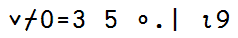0  0  1  0  1  1  0  0  1

4) since this array has the same length as our input, we can just multiply the two together!

0  0  3  0  5  6  0  0  9

5) and finally reduce over this array using addition and voila!